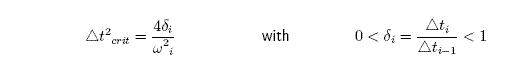# Time integration

## Equation of Motion

Single degree of freedom system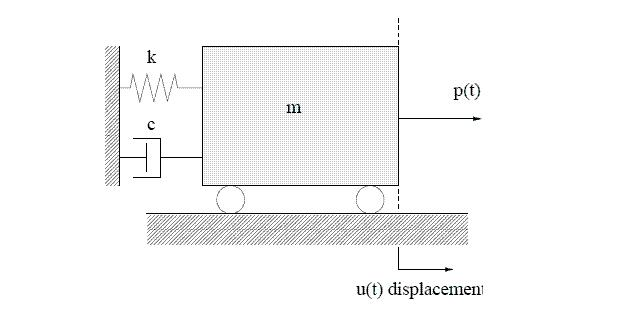Forces acting on mass m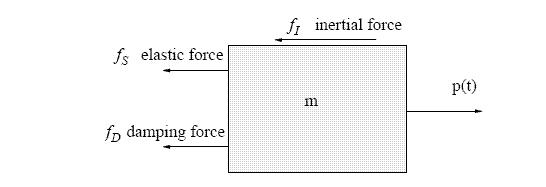Equilibrium – d Alembert s principle :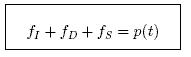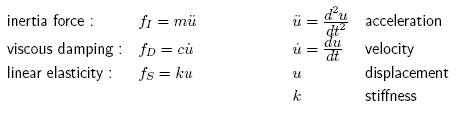Equation of motion for linear elasticity :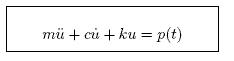linear ordinary d.e.

Nonlinear case :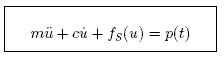nonlinear ordinary d.e.

Analytical solutions of linear ordinary di erential equations are available.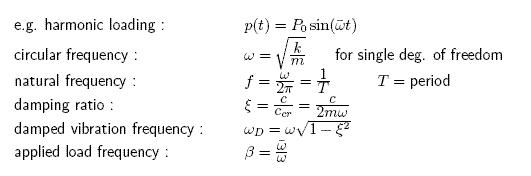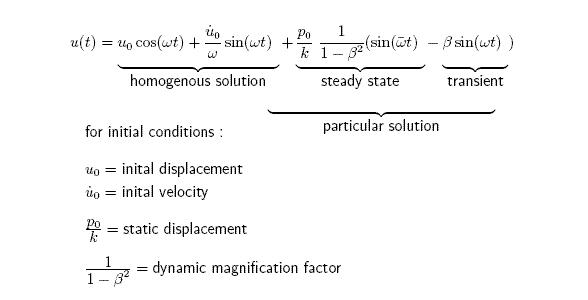## Direct Integration of Equation of Motion

• For nonlinear problems only numerical solutions are possible.
• Focus is on explicit methods, in particular Central Difference method.
• LS-DYNA uses a modification of the central di erence time integration.
• Central difference scheme is an explicit method.
• For explicit schemes the equation of motion is evaluated at the old time step tn, whereas implicit methods use the equation of motion at the new time step tn+1.

## Central Difference

• discretization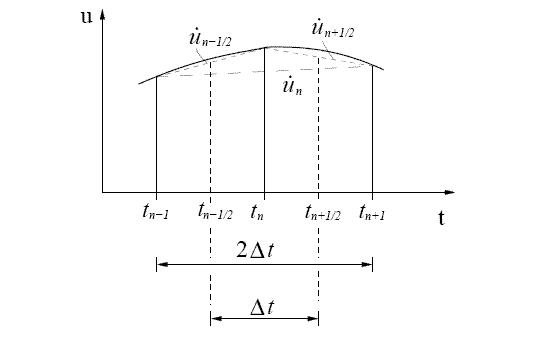• difference formula :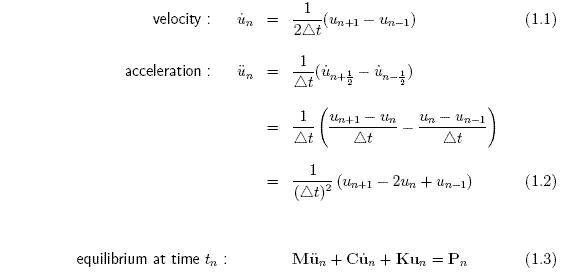Substitute equations (??) and (??) into (??) :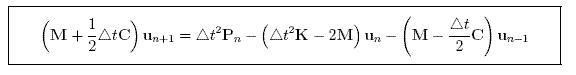• For lumped mass and damping the matrices M are diagonal.• Inversion of diagonal matrices M and C is trivial.• At timer t = 0 we have initial conditions u0 and &u-odot0. From equilibrium we find ü0. From equation (??) and (??) :• The central di erence scheme is conditionally stable, i.e. the size of the time step is limited.

## Stability of the Central Difference Scheme

Uncouple the system of linear equations of motion into the modal equations.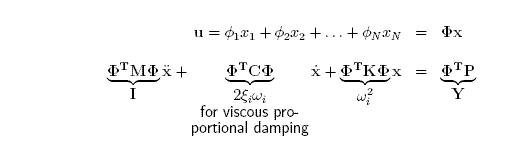Φ … modal matrix with M-orthonormalized eigenvectors stored in columns N uncoupled equations of motion with generalized displacements χ :central differences :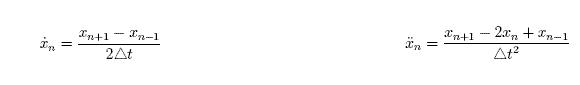Substitute xn and xn into equation of motion () at time t^n* :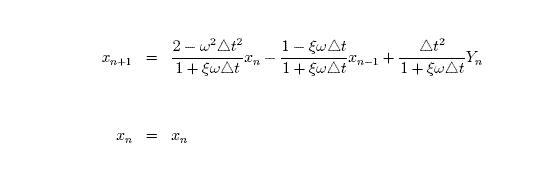In matrix form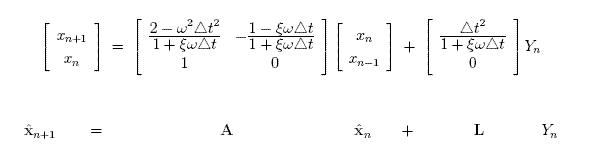A… time integration operator for discrete

For m-time steps and L = 0Spectral decomposition of A :P … orthonormal matrix; contains eigenvectors of A

J … Jordan form; eigenvalues λi of A are stored on diagonal

spectral radius = ρ(A) = largest eigenvalue of A = max ((diag(J))Eigenvalues of A for the undamped equation of motion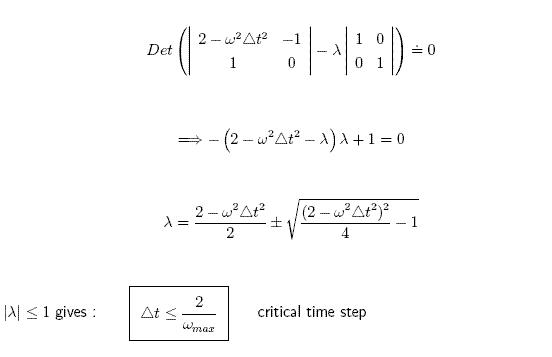For the damped equation of motion :Damping reduces the critical time step.

For varying time step sizes :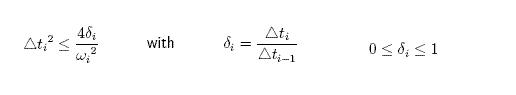• The time integration is stable, if the time step size decreases.
• The time step is bounded by the largest natural frequency of the structure.
• For shells: bending and membrane modes are present the frequency of the membrane mode usually limits the critical time step, since membrane stiffness is much larger than bending stiffness

## Critical time step of a rog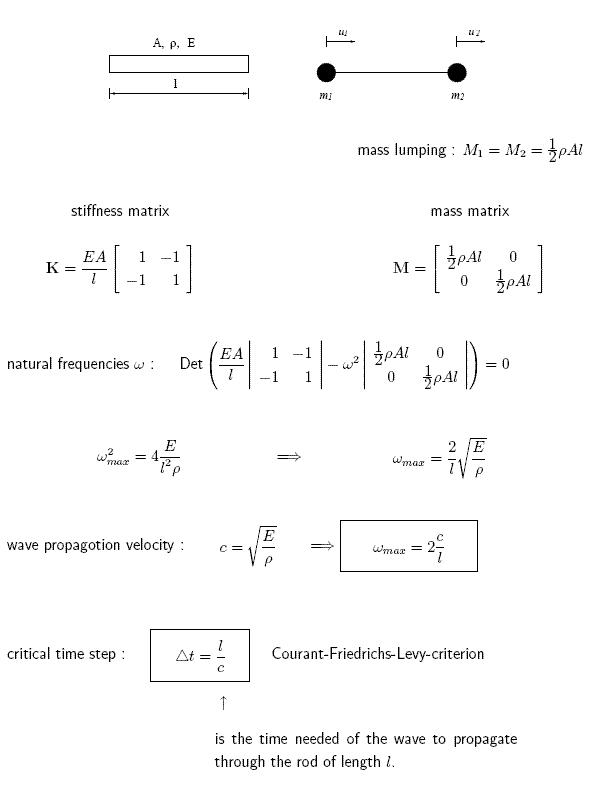## Time Integration in LS-DYNA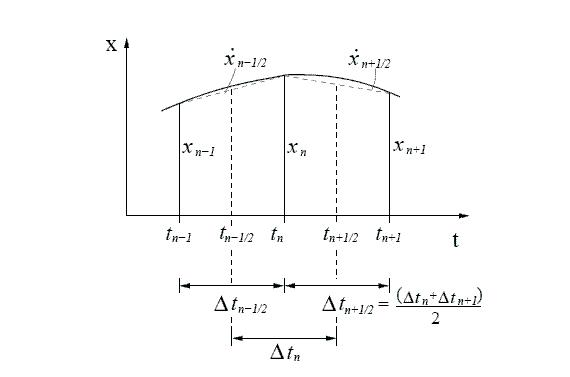• discretization In LS-DYNA actual geometry x is used instead of displacements. Thus x replaces u.
• difference formula :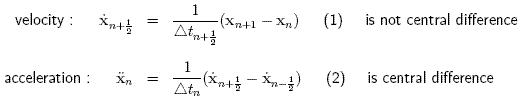equation of motion at time t^n for the nonlinear case:(asynchronous damping)

assumption :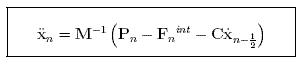update of accelerations

update formulas for velocities and displacements:

From (2):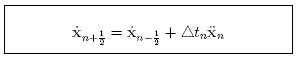From (1):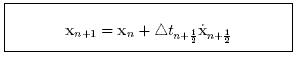Remarks :

• starting procedure for first time step with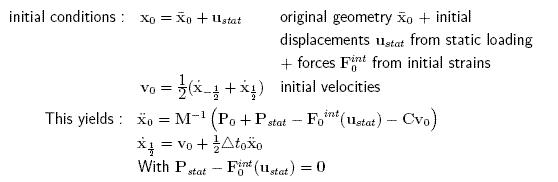• Standard central di erence method approximates time step limit for LS-DYNA time integration scheme.
• No stability proofs are available for time integration of nonlinear problems. Default in LS-DYNA :• critical time step for varying time increments